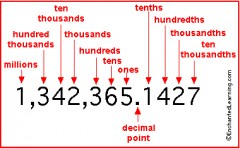# How Do You Change A Decimal Into A Mixed Number In Lowest Terms?

12.38
thanked the writer.
2 7/0
thanked the writer.
7.79
thanked the writer.
Change 2.7 into a fraction
thanked the writer.
Give me a number
thanked the writer.
2.45
thanked the writer.
You multiply the decimal  numbers and and half
thanked the writer.
2.4=2/4,hope it help,from carey
thanked the writer.You make use of the place values of the digits, and you reduce the resulting fraction in the usual way. Example: 1.8 = 1 8/10 = 1 4/5
thanked the writer.
Convert 5.25 into a mixed number the answer has to be 5 1/4, 5 3/4, or 5 1/2
thanked the writer.
It's very simple actually. Take the decimal integers as numerator and then place a digit 1 under the decimal point and place zeros followed by 1 for each digit in the numerator. After, simplifying the fraction you will have the required fraction. For example, if I am to convert 0.0025 into a fraction, the proceeding operation will be :-
=> 0.0025
=> 0025/10000
=> 25/10000
=> 5/1000
=> 1/200
thanked the writer.
2 7/10
thanked the writer.
6.025 into a mixed number
thanked the writer.
96.1 into a mixed number
thanked the writer.
7.79 miles
thanked the writer.
Convert 7.79 in a mixed number
thanked the writer.
6.025
thanked the writer.
You need math to do all your works!
thanked the writer.
73.000 to mixed number
thanked the writer.
33.313 into a mixed number
thanked the writer.
Ok I havent learned about intergers r wateva yet brake it down to 6th grade form!!
thanked the writer.
The integer part of the decimal is the integer part of the mixed number. The part of the decimal to the right of the decimal point can be written as a fraction based on the place values of the digits.

8.57 = 8 57/100    (this fraction cannot be reduced)

After you have the fraction, you can reduce it, if possible.

6.125 = 6 125/1000 = 6 1/8
thanked the writer.